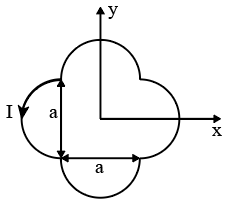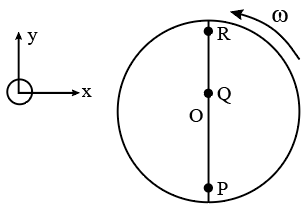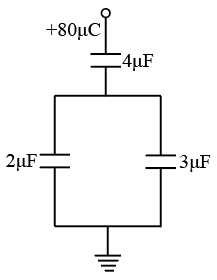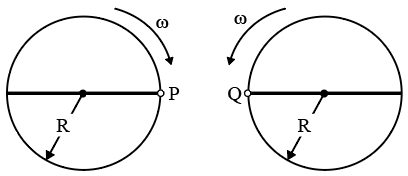Instructions

For the following questions answer them individually

Question 1

# A loop carrying current I lies in the x-y plane as shown in the figure. the unit vector $$\hat{k}$$ is coming out of the plane of the paper. the magnetic moment of the current loop is :Question 2

# A thin uniform cylindrical shell, closed at both ends, is partially filled with water. It is floating vertically in water in half-submerged state. If $$\rho_c$$ is the relative density of the material of the shell with respect to water, then the correct statement is that the shell isQuestion 3

# An infinitely long hollow conducting cylinder with inner radius R/2 and outer radius R carries a uniform current density along is length. Themagnitude of themagnetic field, $$\mid \overrightarrow{B} \mid$$ as a function of the radial distance r from the axis is best represented by :Question 4

# Consider a disc rotating in the horizontal plane with a constant angular speed $$\omega$$ about its centre O. The disc has a shaded region on one side of the diameter and an unshaded region on the other side as shown in the figure. When the disc is in the orientation as shown, two pebbles P and Q are simultaneously projected at an angle towards R. The velocity of projection is in the y-z plane and is same for both pebbles with respect to the disc. Assume that (i) they land back on the disc before the disc completed $$\frac{1}{8}$$ rotation. (ii) their range is less than half disc radius, and (iii) $$\omega$$ remains constant throughout . ThenQuestion 5

# A student is performing the experiment of Resonance Column. The diameter of the column tube is 4cm . The distance frequency of the tuning for k is 512 Hz. The air temperature is $$38^\circ C$$ in which the speed of sound is 336 m/s. The zero of the meter scale coincides with the top and of the Resonance column. When first resonance occurs, the reading of the water level in the column isQuestion 6

# In the given circuit, a charge of +80 $$\mu C$$ is given to the upper plate of the $$4 \mu F$$ capacitor. Then in the steady state, the charge on the upper plate of the $$3 \mu F$$ capacitor is :Question 7

# Two identical discs of same radius R are rotating about their axes in opposite directions with the same constant angular speed $$\omega$$. The disc are in the same horizontal plane. At time t = 0 , the points P and Q are facing each other as shown in the figure. The relative speed between the two points P and Q is $$v_r$$. as function of times best represented byQuestion 8

# Two moles of ideal helium gas are in a rubber balloon at $$30^\circ C$$ .The balloon is fully expandable and can be assumed to require no energy in its expansion. The temperature of the gas in the balloon is slowly changed to $$35^\circ C$$.The amount of heat required in raising the temperature is nearly (take R = 8.31 J/mol.K)Instructions

The $$\beta$$- decay process, discovered around 1900, is basically the decay of a neutron (n), In the laboratory, a proton (p) and an electron $$(e^{-})$$ are observed as the decay products of the neutron. therefore, considering the decay of a neutron as a tro-body dcay process, it was predicted theoretically that thekinetic energy of the electron should be a constant. But experimentally, it was observed that the electron kinetic energy has a continuous spectrum. Considering a three-body decay process, i.e. $$n \rightarrow p + e^{-} + \overline{v_e}$$, around 1930, pauli explained the observed electron energy spectrum. Assuming the anti-neutrino $$(\overline{v_e})$$ to be massless and possessing negligible energy, and neutron to be at rest, momentum and energy conservation principles are applied. From this calculation, themaximum kinetic energy of the lectron is $$0.8 \times 10^6$$ eV. The kinetic energy carried by the proton is only the recoil energy.

Question 9

# What is the maximum energy of the anti-neutrino ?Question 10

# If the anti-neutrino had amass of 3 eV/c$$^2$$ (where c is the speed of light) instead of zeromass, what should be the range of the kinetic energy, K, of the electron ?OR